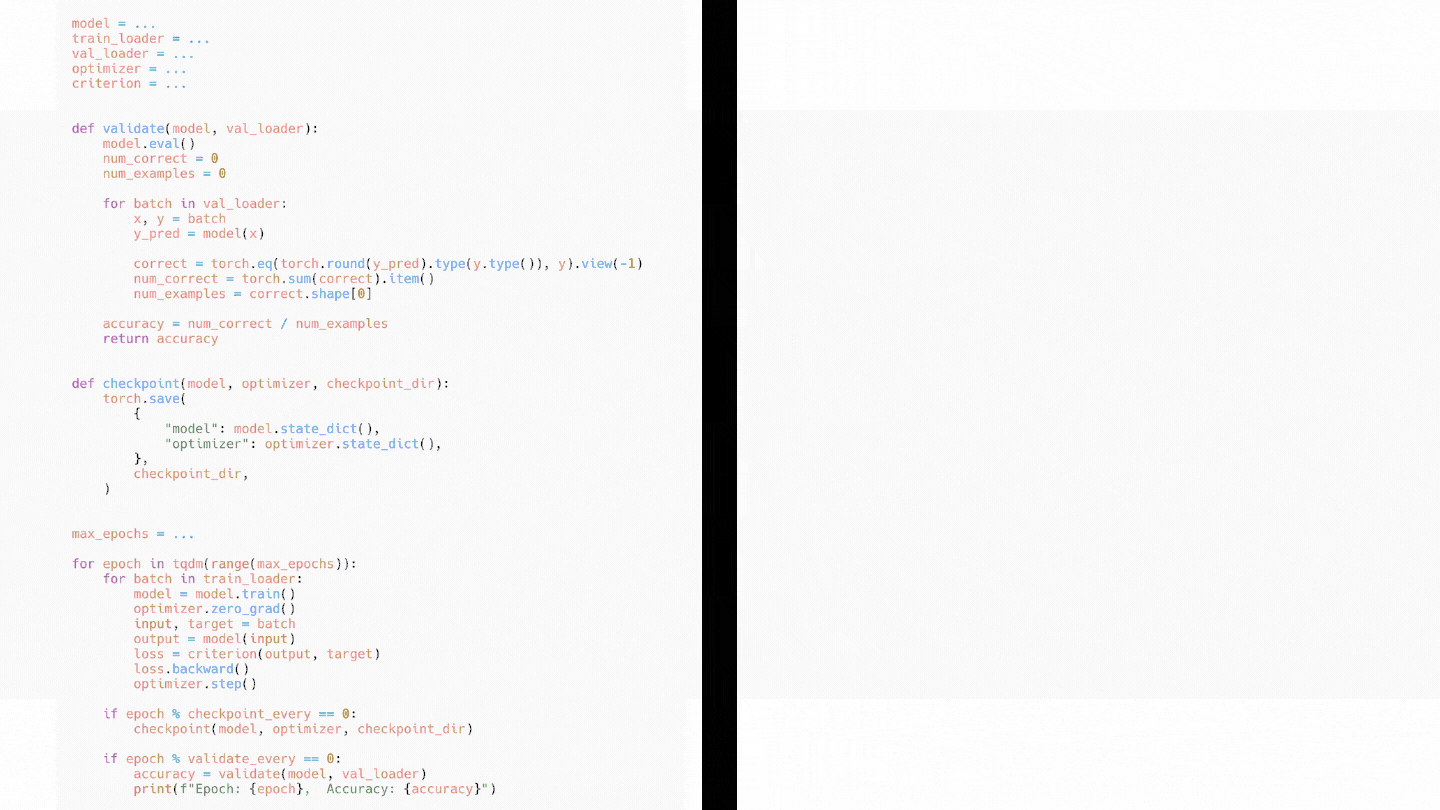# How to convert pure PyTorch code to Ignite

In this guide, we will show how PyTorch code components can be converted into compact and flexible PyTorch-Ignite code.Since Ignite focuses on the training and validation pipeline, the code for models, datasets, optimizers, etc will remain user-defined and in pure PyTorch.

``````model = ...
optimizer = ...
criterion = ...
``````

## Training Loop to `trainer`

A typical PyTorch training loop processes a single batch of data, passes it through the `model`, calculates `loss`, etc as below:

``````for batch in train_loader:
model.train()
inputs, targets = batch
outputs = model(inputs)
loss = criterion(outputs, targets)
loss.backward()
optimizer.step()
``````

To convert the above code into Ignite we need to move the code or steps taken to process a single batch of data while training under a function (`train_step()` below). This function will take `engine` and `batch` (current batch of data) as arguments and can return any data (usually the loss) that can be accessed via `engine.state.output`. We pass this function to `Engine` which creates a `trainer` object.

``````from ignite.engine import Engine

def train_step(engine, batch):
model.train()
inputs, targets = batch
outputs = model(inputs)
loss = criterion(outputs, targets)
loss.backward()
optimizer.step()
return loss.item()

trainer = Engine(train_step)
``````

There are other helper methods that directly create the `trainer` object without writing a custom function for some common use cases like supervised training and truncated backprop through time.

## Validation Loop to `evaluator`

The validation loop typically makes predictions (`y_pred` below) on the `val_loader` batch by batch and uses them to calculate evaluation metrics (Accuracy, Intersection over Union, etc) as below:

``````model.eval()
num_correct = 0
num_examples = 0

x, y = batch
y_pred = model(x)

correct = torch.eq(torch.round(y_pred).type(y.type()), y).view(-1)
num_correct = torch.sum(correct).item()
num_examples = correct.shape
print(f"Epoch: {epoch},  Accuracy: {num_correct / num_examples}")
``````

We will convert this to Ignite in two steps by separating the validation and metrics logic.

We will move the model evaluation logic under another function (`validation_step()` below) which receives the same parameters as `train_step()` and processes a single batch of data to return some output (usually the predicted and actual value which can be used to calculate metrics) stored in `engine.state.output`. Another instance (called `evaluator` below) of `Engine` is created by passing the `validation_step()` function.

``````def validation_step(engine, batch):
model.eval()
x, y = batch
y_pred = model(x)

return y_pred, y

evaluator = Engine(validation_step)
``````

Similar to the training loop, there are helper methods to avoid writing this custom evaluation function like `create_supervised_evaluator`.

Note: You can create different evaluators for training, validation, and testing if they serve different purposes. A common practice is to have two separate evaluators for training and validation, since the results of the validation evaluator are helpful in determining the best model to save after training.

## Switch to built-in Metrics

Then we can replace the code for calculating metrics like accuracy and instead use several out-of-the-box metrics that Ignite provides or write a custom one (refer here). The metrics will be computed using the `evaluator`’s output. Finally, we attach these metrics to the `evaluator` by providing a key name (“accuracy” below) so they can be accessed via `engine.state.metrics[key_name]`.

``````from ignite.metrics import Accuracy

Accuracy().attach(evaluator, "accuracy")
``````

## Organizing code into Events and Handlers

Next, we need to identify any code that is triggered when an event occurs. Examples of events can be the start of an iteration, completion of an epoch, or even the start of backprop. We already provide some predefined events (complete list here) however we can also create custom ones (refer here). We move the event-specific code to different handlers (named functions, lambdas, class functions) which are attached to these events and executed whenever a specific event happens. Here are some common handlers:

### Running `evaluator`

We can convert the code that runs the `evaluator` on the training/validation/test dataset after `validate_every` epoch:

``````if epoch % validate_every == 0:
# Validation logic
``````

by attaching a handler to a built-in event `EPOCH_COMPLETED` like:

``````from ignite.engine import Events

validate_every = 10

@trainer.on(Events.EPOCH_COMPLETED(every=validate_every))
def run_validation():
``````

### Logging metrics

Similarly, we can log the validation metrics in another handler or combine it with the above handler.

``````@trainer.on(Events.EPOCH_COMPLETED(every=validate_every))
def log_validation():
metrics = evaluator.state.metrics
print(f"Epoch: {trainer.state.epoch},  Accuracy: {metrics['accuracy']}")
``````

### Progress Bar

We use a built-in wrapper around `tqdm` called `ProgressBar()`.

``````from ignite.contrib.handlers import ProgressBar

ProgressBar().attach(trainer)
``````

### Checkpointing

Instead of saving all models after `checkpoint_every` epoch:

``````if epoch % checkpoint_every == 0:
checkpoint(model, optimizer, "checkpoint_dir")
``````

we can smartly save the best `n_saved` models (depending on `evaluator.state.metrics`), and the state of `optimizer` and `trainer` via the built-in `Checkpoint()`.

``````from ignite.handlers import Checkpoint

checkpoint_every = 5
checkpoint_dir = ...

checkpointer = Checkpoint(
to_save={'model': model, 'optimizer': optimizer, 'trainer': trainer},
save_handler=checkpoint_dir, n_saved=2
)
Events.EPOCH_COMPLETED(every=checkpoint_every), checkpointer
)
``````

## Run for a number of epochs

``````max_epochs = ...
we begin training on `train_loader` via:
``````trainer.run(train_loader, max_epochs)